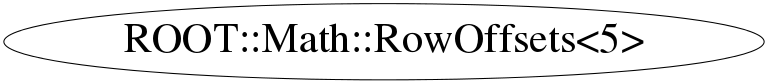# class ROOT::Math::RowOffsets<5>


MatRepStd
Standard Matrix representation for a general D1 x D2 matrix.
This class is itself a template on the contained type T, the number of rows and the number of columns.
Its data member is an array T[nrows*ncols] containing the matrix data.
The data are stored in the row-major C convention.
For example, for a matrix, M, of size 3x3, the data \f$\left[a_0,a_1,a_2,.......,a_7,a_8 \right] \f$d are stored in the following order:
\f[
M = \left( \begin{array}{ccc}
a_0 & a_1 & a_2  \\
a_3 & a_4  & a_5  \\
a_6 & a_7  & a_8   \end{array} \right)
\f]

@ingroup MatRep



## Function Members (Methods)

public:
 ~RowOffsets<5>() int apply(unsigned int i) const int operator()(unsigned int i, unsigned int j) const ROOT::Math::RowOffsets<5>& operator=(const ROOT::Math::RowOffsets<5>&) ROOT::Math::RowOffsets<5>& operator=(ROOT::Math::RowOffsets<5>&&) ROOT::Math::RowOffsets<5> RowOffsets<5>() ROOT::Math::RowOffsets<5> RowOffsets<5>(const ROOT::Math::RowOffsets<5>&) ROOT::Math::RowOffsets<5> RowOffsets<5>(ROOT::Math::RowOffsets<5>&&)

## Data Members

public:
 int fOff

## Class Charts## Function documentation

const T& operator()(unsigned int i, unsigned int j) const
T apply(unsigned int i) const
{ return fArray[i]; }
MatRepStd<T, D1, D2>& operator=(const R& rhs)
MatRepSym<T, D>& operator=(const R&)
         assignment : only symmetric to symmetric allowed